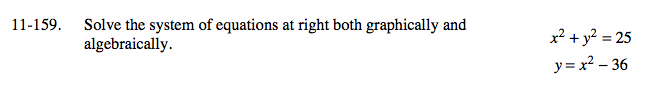Home > A2C > Chapter 11 > Lesson 11.3.3 > Problem11-159

11-159.

Solve the system of equations below both graphically and algebraically. Homework Help ✎
x2 + y2 = 25
y = x2 − 36Note that based on the graph there will be no real solutions.

Substitute for x2 in the first equation and solve for y.